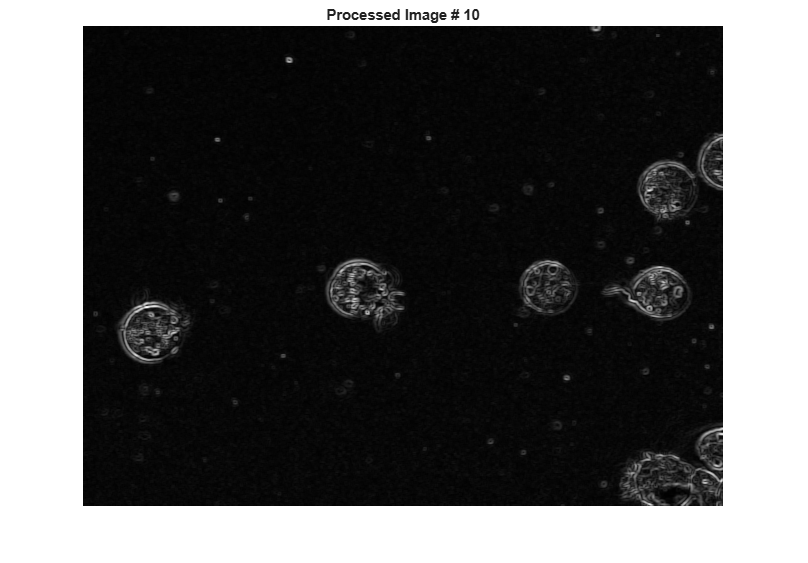Perform an Operation on a Sequence of Images

This example shows how to perform an operation on a sequence of images. The example creates an array of images and passes the entire array to the stdfilt function to perform standard deviation filtering on each image in the sequence.

Create an array of file names.

fileFolder = fullfile(matlabroot,'toolbox','images','imdata');
dirOutput = dir(fullfile(fileFolder,'AT3_1m4_*.tif'));
fileNames = {dirOutput.name}'
numFrames = numel(fileNames)
fileNames =

10x1 cell array

{'AT3_1m4_01.tif'}
{'AT3_1m4_02.tif'}
{'AT3_1m4_03.tif'}
{'AT3_1m4_04.tif'}
{'AT3_1m4_05.tif'}
{'AT3_1m4_06.tif'}
{'AT3_1m4_07.tif'}
{'AT3_1m4_08.tif'}
{'AT3_1m4_09.tif'}
{'AT3_1m4_10.tif'}

numFrames =

10

Preallocate an m -by- n -by- p array and read images into the array.

sequence = zeros([size(I) numFrames],class(I));
sequence(:,:,1) = I;

for p = 2:numFrames
end

Process each image in the sequence, performing standard deviation filtering. Note that, to use stdfilt with an image sequence, you must specify the nhood argument, passing a 2-D neighborhood.

sequenceNew = stdfilt(sequence,ones(3));

View each input image followed by its processed image.

figure;
for k = 1:numFrames
imshow(sequence(:,:,k));
title(sprintf('Original Image # %d',k));
pause(1);
imshow(sequenceNew(:,:,k),[]);
title(sprintf('Processed Image # %d',k));
pause(1);
end